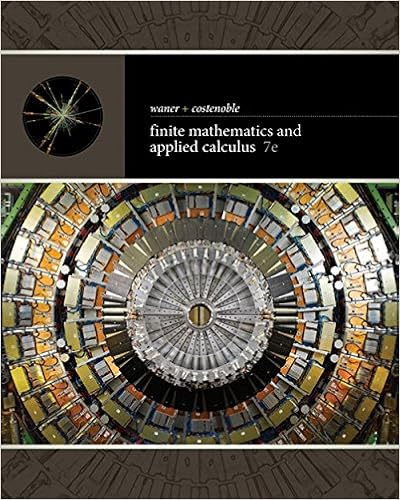# Quantity mc d c b a k m h g f l j e i atc avc price

• Homework Help
• 13
• 93% (126) 117 out of 126 people found this document helpful

This preview shows page 6 - 9 out of 13 pages.

##### We have textbook solutions for you!
The document you are viewing contains questions related to this textbook.The document you are viewing contains questions related to this textbook.
Chapter 2 / Exercise 53
Finite Mathematics and Applied Calculus
Costenoble/WanerExpert Verified
Quantity MC D C B A K M H G F L J E I ATC AVC Price & cost (\$/unit) 1,000 \$100 0
Quantity MC Price & cost (\$/unit) ATC AVC 11 \$10.25 9 7.50 7 0 Goolsbee1e_Solutions_Manual_Ch08.indd 98 Goolsbee1e_Solutions_Manual_Ch08.indd 98 11/15/12 3:09 PM 11/15/12 3:09 PM
##### We have textbook solutions for you!
The document you are viewing contains questions related to this textbook.The document you are viewing contains questions related to this textbook.
Chapter 2 / Exercise 53
Finite Mathematics and Applied Calculus
Costenoble/WanerExpert Verified
Supply in a Competitive Market Chapter 8 99 Solution If the price is \$9, the price at the optimum quantity (where MC is equal to 9) would fall in between AVC and ATC . In that case, operating profit ( TR minus VC ) would be positive in the short run and en- able the owner to cover at least part of the fixed cost. In that case, the owner should produce in the short run. However, since profit is negative overall ( TR TC < 0), the owner should shut down production in the long run. 11. Marty sells flux capacitors in a perfectly competitive market. His marginal cost is given by MC = Q . Thus, the first capacitor Marty produces has a marginal cost of \$1, the second has a marginal cost of \$2, and so on. a. Draw a diagram showing the marginal cost of each unit that Marty produces. b. If flux capacitors sell for \$2, determine the profit-maximizing quantity for Marty to produce. c. Repeat part (b) for \$3, \$4, and \$5. d. The supply curve for a firm traces out the quantity that firm will produce and offer for sale at various prices. Assuming that the firm chooses the quantity that maximizes its profits [you solved for these in (b) and (c)], draw another diagram showing the supply curve for Marty’s flux capacitors. e. Compare the two diagrams you have drawn. What can you say about the supply curve for a competitive firm? Quantity of flux capacitors Price & cost (\$/capacitor) \$5 0 MC = Q 5 Quantity of flux capacitors Price & cost (\$/capacitor) \$5 0 Supply 5
Goolsbee1e_Solutions_Manual_Ch08.indd 99 Goolsbee1e_Solutions_Manual_Ch08.indd 99 11/15/12 3:09 PM 11/15/12 3:09 PM
100 Part 3 Markets and Prices Solution Solution 12. Consider the graph on the right, which depicts the cost curves of a perfectly competitive seller of potatoes. Potatoes currently sell for \$3 per pound. a. To maximize profit, how many pounds of potatoes should this seller produce? Suppose that the potato grower’s bank ratchets up the interest rate applicable to the grower’s adjustable-rate mortgage loan. This increases the size of the potato grower’s monthly mortgage payment.
•••